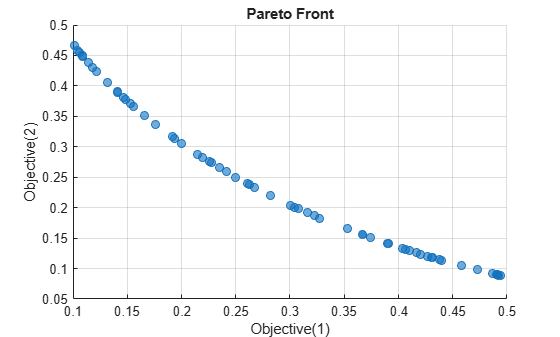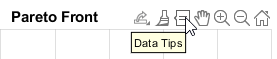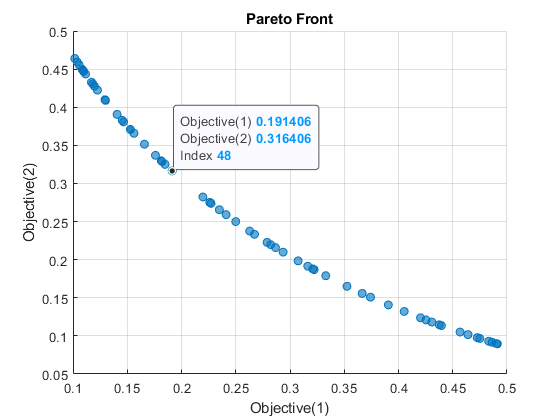# OptimizationValues

Values for optimization problems

Since R2022a

## Description

An `OptimizationValues` object holds values used by and returned from `solve` for multiobjective problems. The object also holds starting values for the `x0` argument for solvers that accept multiple start points.

## Creation

The `solve` function returns a vector of `OptimizationValues` objects as the solution to a multiobjective problem.

Create an `OptimizationValues` object for a start point `x0` by using the `optimvalues` function.

## Properties

expand all

Typically, `OptimizationValues` properties are dynamic: they are the names of the optimization variables, objective function or functions, and constraints.

However, you can also have unnamed objective functions or constraints. For those cases, `OptimizationValues` assigns the following properties.

Objective function values, returned or specified as a real array.

Data Types: `double`

Constraint values, returned or specified as a real array.

Data Types: `double`

## Object Functions

 `paretoplot` Pareto plot of multiobjective values

## Examples

collapse all

Create and solve a multiobjective problem using optimization variables.

```x = optimvar("x",LowerBound=-3,UpperBound=3); prob = optimproblem; prob.Objective = [x^2;(x-1)^2]; % Tradeoff region between x = 0 and x = 1 prob.Constraints.con1 = x^2 <= 1/2; % Demonstrate constraints prob.Constraints.con2 = x^2 >= 1/10; % Second constraint rng default % For reproducibility [sol,fval,exitflag,output] = solve(prob,Solver="paretosearch")```
```Solving problem using paretosearch. Pareto set found that satisfies the constraints. Optimization completed because the relative change in the volume of the Pareto set is less than 'options.ParetoSetChangeTolerance' and constraints are satisfied to within 'options.ConstraintTolerance'. ```
```sol = 1x60 OptimizationValues vector with properties: Variables properties: x: [0.7027 0.7014 0.3635 0.3491 0.5723 0.3177 0.4634 0.4400 0.6379 0.6402 0.3750 0.6057 0.6565 0.5483 0.6455 0.5518 0.3760 0.3232 0.6768 0.6357 0.5625 0.3952 0.3382 0.3857 0.5677 0.5170 0.5107 0.6241 0.6615 0.3295 0.6875 0.4473 0.6630 ... ] Objective properties: Objective: [2x60 double] Constraints properties: con1: [-0.0063 -0.0081 -0.3679 -0.3781 -0.1725 -0.3991 -0.2853 -0.3064 -0.0931 -0.0902 -0.3594 -0.1332 -0.0690 -0.1994 -0.0833 -0.1955 -0.3586 -0.3955 -0.0420 -0.0959 -0.1836 -0.3438 -0.3856 -0.3512 -0.1777 -0.2327 -0.2392 -0.1105 -0.0625 ... ] con2: [-0.3937 -0.3919 -0.0321 -0.0219 -0.2275 -9.3572e-04 -0.1147 -0.0936 -0.3069 -0.3098 -0.0406 -0.2668 -0.3310 -0.2006 -0.3167 -0.2045 -0.0414 -0.0045 -0.3580 -0.3041 -0.2164 -0.0562 -0.0144 -0.0488 -0.2223 -0.1673 -0.1608 -0.2895 ... ] ```
```fval = 2×60 0.4937 0.4919 0.1321 0.1219 0.3275 0.1009 0.2147 0.1936 0.4069 0.4098 0.1406 0.3668 0.4310 0.3006 0.4167 0.3045 0.1414 0.1045 0.4580 0.4041 0.3164 0.1562 0.1144 0.1488 0.3223 0.2673 0.2608 0.3895 0.4375 0.1086 0.4727 0.2001 0.4395 0.2261 0.1658 0.4862 0.4270 0.3525 0.1057 0.2628 0.2197 0.4902 0.1760 0.3665 0.2411 0.1092 0.4911 0.1914 0.1182 0.3742 0.0884 0.0892 0.4051 0.4237 0.1829 0.4655 0.2880 0.3136 0.1311 0.1295 0.3906 0.1555 0.1180 0.2040 0.1257 0.2009 0.3893 0.4580 0.1045 0.1327 0.1914 0.3658 0.4380 0.3773 0.1869 0.2333 0.2394 0.1413 0.1146 0.4496 0.0977 0.3055 0.1136 0.2751 0.3514 0.0916 0.1201 0.1650 0.4555 0.2375 0.2822 0.0899 0.3369 0.1557 0.2591 0.4483 0.0895 0.3164 0.4307 0.1508 ```
```exitflag = SolverConvergedSuccessfully ```
```output = struct with fields: iterations: 20 funccount: 380 volume: 1.8611 averagedistance: 0.0101 spread: 0.3067 maxconstraint: 0 message: 'Pareto set found that satisfies the constraints. ...' rngstate: [1x1 struct] solver: 'paretosearch' ```

The `paretosearch` solver converges in 16 iterations to a feasible solution. Plot the solution.

`paretoplot(sol)`Choose an arbitrary point to examine in the plot using Data Tips:The pictured point is at index 48. Examine solution 48.

`arbitrarysol = sol(48)`
```arbitrarysol = OptimizationValues with properties: Variables properties: x: 0.4375 Objective properties: Objective: [2x1 double] Constraints properties: con1: -0.3086 con2: -0.0914 ```

The constraint values are negative, meaning the pictured point is feasible.

`arbitrarysol.Objective`
```ans = 2×1 0.1914 0.3164 ```

The objective values match the values in the Data Tips.

## Limitations

• `OptimizationValues` objects support horizontal concatenation only. In other words, you can have only row vectors of `OptimizationValues` objects.

## Version History

Introduced in R2022a